# Time series modeling in numpyro using MCMC

Hi all,

I am trying to fit a mutivariate time series model in numpyro. The model is a state-space model with AR(1). The data consists of two time series with missing values. I used the non-centered parametrization and jax.lax.scan as in Using lax.scan for time series in NumPyro. I am doing inference using NUTS. The generative process and the code of the model are the following: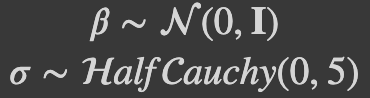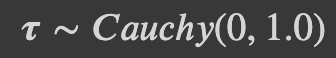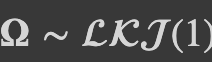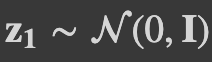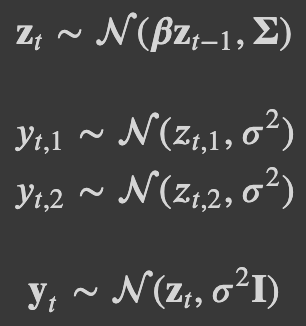``````# Definition of the function for the jax.lax.scan
def f(carry, noise_t):
beta, z_prev = carry
z_t = beta*z_prev + noise_t
z_prev = z_t
return (beta, z_prev), z_t

def model(T, T_forecast, obs1=None, ix_mis1=None, ix_obs1=None, obs2=None, ix_mis2=None, ix_obs2=None):
"""
Prior for the betas, sigma and first state
"""
beta = numpyro.sample("beta", numpyro.distributions.Normal(jnp.zeros(2), jnp.ones(2)))
sigma = numpyro.sample("sigma", numpyro.distributions.HalfCauchy(jnp.ones(2)))
z_prev = numpyro.sample("z_prev", numpyro.distributions.Normal(jnp.zeros(2), jnp.ones(2)))

"""
Prior for the LKJ including a tau and the sampling noise
"""
tau = numpyro.sample("tau", numpyro.distributions.HalfCauchy(jnp.ones(2)))
L_Omega = numpyro.sample("L_Omega", numpyro.distributions.LKJCholesky(dimension=2, concentration=1.))
Sigma_lower = jnp.matmul(jnp.diag(jnp.sqrt(tau)), L_Omega)
noises = numpyro.sample("noises",
fn=dist.MultivariateNormal(loc=jnp.zeros(2), scale_tril=Sigma_lower),
sample_shape=(T+T_forecast-1,))

"""
Propagate the dynamics forward using jax.lax.scan
"""
carry = (beta, z_prev)
z_collection = [z_prev]

updated_carry, zs = lax.scan(f=f, init=carry, xs=noises, length=T+T_forecast-1)

z_collection = jnp.concatenate((jnp.array(z_collection), zs), axis=0)

"""
Sample the observed y (y_obs) and missing y (y_mis)
"""
y1_obs  = numpyro.sample("y1_obs", numpyro.distributions.Normal(z_collection[ix_obs1, 0], sigma), obs=obs1)
y1_mis  = numpyro.sample("y1_mis", numpyro.distributions.Normal(z_collection[ix_mis1, 0], sigma))
y1_pred = numpyro.sample("y1_pred", numpyro.distributions.Normal(z_collection[T:, 0], sigma))

y2_obs  = numpyro.sample("y2_obs", numpyro.distributions.Normal(z_collection[ix_obs2, 1], sigma), obs=obs2)
y2_mis  = numpyro.sample("y2_mis", numpyro.distributions.Normal(z_collection[ix_mis2, 1], sigma))
y2_pred = numpyro.sample("y2_pred", numpyro.distributions.Normal(z_collection[T:, 1], sigma))
``````

I have two questions about the model:

1. Why does the model “learn” a distribution for each of the noises? I would expect the posterior of noises to be just standard gaussians. Almost all of them are, but some are clearly not. If the noise is “exogenous” why can’t I input just numpy samples from a standard gaussian?
2. When I run the model without the predictions (y1_pred and y2_pred) the model runs relatively smoothly (still with the problem in 1) but I get sensible results), but whenever I include the forecasting part the model collapses. That is, the posterior become single values sampled over and over with n_eff=0.5 for all of them.

Thank you,
Sergio

Hi @chechgm, about 1., I guess you want to marginalize the latent variable `z`? If it is the case, then you might want to use Pyro GaussianHMM instead (currently, that class does not support missing observations - though it is doable).

About 2., you can use Predictive for forecasting. You can distinguish training and forecasting using a flag as in this example. In addition, I think you can remove the missing variables `y*_mis` because it does not help for inference. If you want to get predictions of those missing values, you can use Predictive after you already get posterior samples using MCMC.

1 Like

1. About 1 maybe the problem is more conceptual than the programming itself. I think I probably don’t understand the point of reparametrization properly. If I am wrong, the point of reparametrization is to express a distribution in different terms so that the inference is easier. The classical example is N(mu, sigma) vs. mu+sigma*N(0,1). What I find puzzling is that in my program, numpyro is learning mu sigma, but also a posterior for N(0,1). Shouldn’t it “stay” as N(0,1) since the information of the inference is contained in mu and sigma? is this (maybe) a problem of identifiability? Do you have any references on the fundamentals of reparametrization?

2. I think using Predictive makes way more sense than the way it is on the code now. I checked the code you sent, but I think that the flag comes from the fact that you have covariates so it is relatively easy to distinguish between train and forecast. In my case I tried:

``````y = jnp.empty((T+T_forecast))
y[:T] = numpyro.sample("y", numpyro.distributions.Normal(z_collection[:T], sigma), obs=obs)
y[T:] = numpyro.sample("y", numpyro.distributions.Normal(z_collection[T:], sigma))
``````

which is fairly similar to the code you sent. However I am getting the following error:

``````TypeError: '<class 'jax.interpreters.xla.DeviceArray'>' object does not support item assignment. JAX arrays are immutable; perhaps you want jax.ops.index_update or jax.ops.index_add instead?
``````

I guess that what I tried is not the way to go. Is there a way around this?

Best,
Sergio

@chechgm Re `reparameterization`: maybe this Stan reference and this paper are helpful.

numpyro is learning mu sigma, but also a posterior for N(0,1)

NUTS will sample all “latent” variables, including the `noises` (this is a latent variable in your model, unless you use Kalman filter - like Pyro GaussianHMM distribution - to marginalize it). This is different from the case you use `mu` and `sigma` in an “observed” node: NUTS will not sample `obs` if its declaration is `numpyro.sample('obs', dist.Normal(mu, sigma), obs=y)`.

About predictive, there are many ways to do. You can define an arg `forecasting_interval` in your model

``````def model(..., forecasting_interval=0):
...
if forecasting_interval > 0:
numpyro.sample("y_forecast", dist.Normal(...))

predictive = Predictive(model, posterior_samples)
y_forecast = predictive(PRNGKey(0), ..., forecasting_interval=10)['y_forecast']
``````

For your model, to sample posterior, you set `forecasting_interval=0` and only include the observed `y*_obs` statement. To get posterior predictive, you can set `obs* = None`. To forecast, you can set `forecasting_interval > 0` and sample `y*_pred`. You can sample `y*_mis` using either `if obs* is None: y_mis = numpyro.sample(...)` or `if forecasting_interval > 0: y_mis = ...`. It is pretty flexible to choose which way you want using model args, as long as you don’t mix `sample` and `predictive/forecasting` steps.

1 Like

@fehiepsi Thank you! I will check the references out!